# Part 2: The basic operations of image processing¶

In the previous notebook, we've seen how to load and edit an image using Python. Let us now present the fundamental operations on which relies every single imaging software:

• truncation,
• subsampling,
• pixelwise mathematics,
• filtering (also known as convolution product).

But first, we need to re-import the necessary modules:

In :
%matplotlib inline
import center_images             # Center our images
import matplotlib.pyplot as plt  # Graphical display
import numpy as np               # Numerical computations


We re-define our custom display routine:

In :
def display(im):  # Define a new Python routine
"""
Displays an image using the methods of the 'matplotlib' library.
"""
plt.figure(figsize=(8,8))                    # Create a square blackboard
plt.imshow(im, cmap="gray", vmin=0, vmax=1)  # Display 'im' using gray colors,
#     from 0 (black) to 1 (white)


And import an axial slice:

In :
I = imread("data/aortes/1.jpg", as_gray=True)  # Import a jpeg image as grayscale array
I = I / 255  # For convenience, we normalize the intensities in the [0,1] range
display(I)   # Let's display our slice: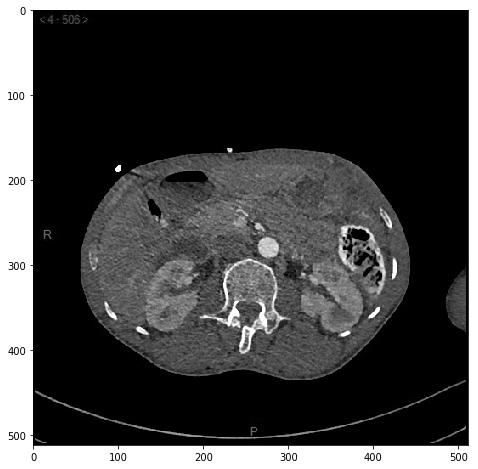### Truncation¶

To truncate (or crop) an image, we simply need to use the "range" syntax presented in the first notebook:

In :
J = I[200:400, 100:300] # Restrict ourselves to a sub-domain of I
display(J)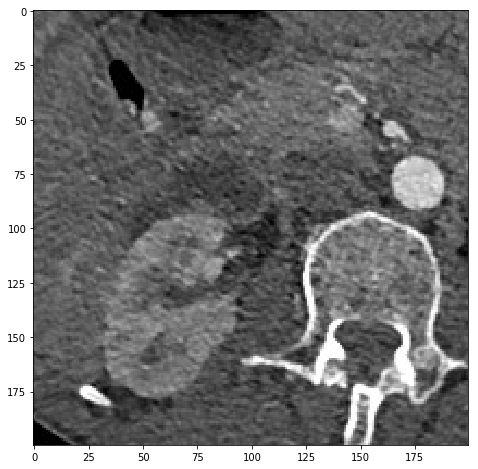### Subsampling¶

Likewise, subsampling can be performed using the stepsize argument:

In :
J = I[::2, ::2] # Only keep "one pixel in two" in both dimensions
display(J)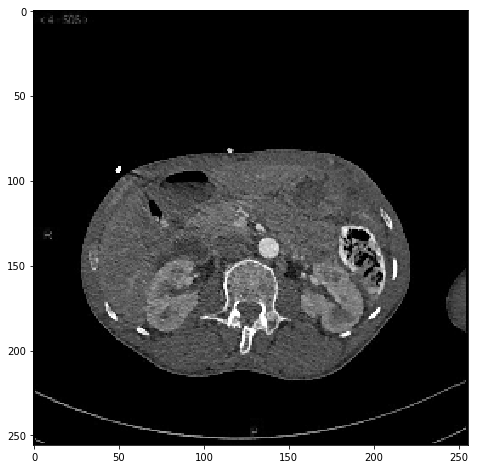In :
J = I[::8, ::8] # Only keep "one pixel in eight" in both dimensions
display(J)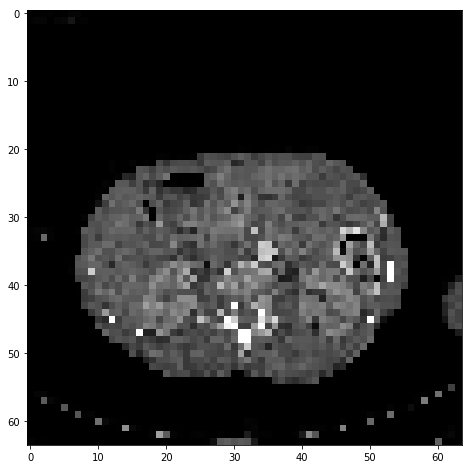### Pixel-wise algebraic operations¶

Python is math-friendly: arbitrary operations can be performed on the variable I and are applied pixel-wise.

In :
J = I / 2  # Halve the intensity across the full image
display(J)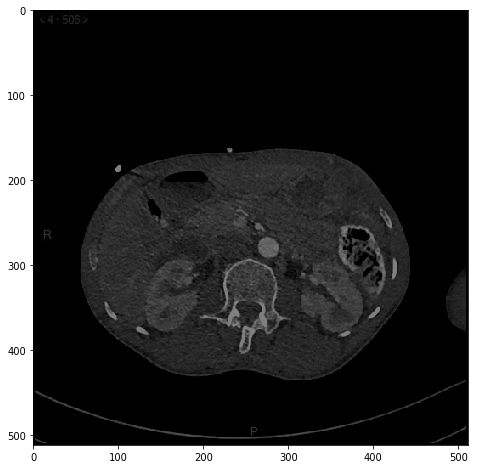In :
J = 2 * I  # Double the intensity - we may saturate in the brighter regions
display(J)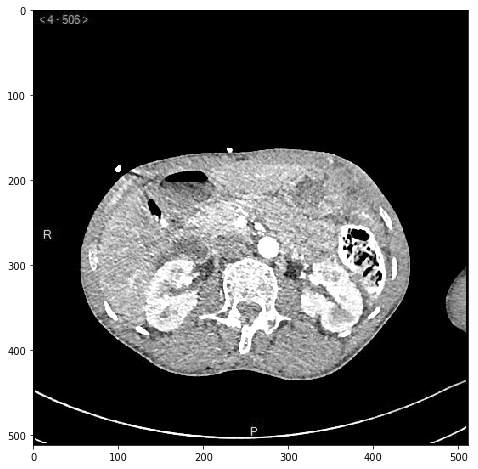In :
J = I**2   # "Square" function, which darkens the grey pixels
display(J)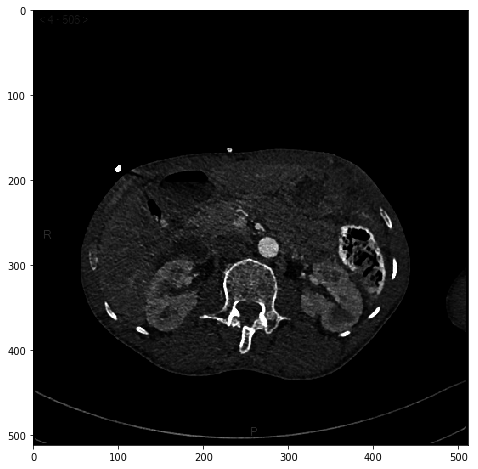In :
J = np.sqrt(I)  # "Square root", which brightens the grey pixels
display(J)